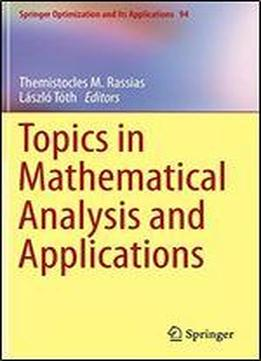# Topics In Mathematical Analysis And Applications (springer Optimization And Its Applications) by Themistocles M. Rassias / 2014 / English / PDF

This volume presents significant advances in a number of theories and problems of Mathematical Analysis and its applications in disciplines such as Analytic Inequalities, Operator Theory, Functional Analysis, Approximation Theory, Functional Equations, Differential Equations, Wavelets, Discrete Mathematics and Mechanics. The contributions focus on recent developments and are written by eminent scientists from the international mathematical community. Special emphasis is given to new results that have been obtained in the above mentioned disciplines in which Nonlinear Analysis plays a central role. Some review papers published in this volume will be particularly useful for a broader readership in Mathematical Analysis, as well as for graduate students. An attempt is given to present all subjects in this volume in a unified and self-contained manner, to be particularly useful to the mathematical community.

views: 227